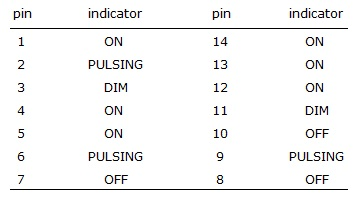# Digital Electronics - Logic Gates

### Exercise :: Logic Gates - General Questions

16.

A logic probe is again applied to the pins of a 7421 IC with the following results. Is there a problem with the circuit and if so, what is the problem?A. Pin 6 should be ON. B. Pin 8 should be ON. C. Pin 8 should be pulsing. D. no problem

Explanation:

No answer description available for this question. Let us discuss.

17.

If a 3-input AND gate has eight input possibilities, how many of those possibilities will result in a HIGH output?

 A. 1 B. 2 C. 7 D. 8

Explanation:

No answer description available for this question. Let us discuss.

18.

The Boolean expression for a 3-input AND gate is ________.

 A. X = AB B. X = ABC C. X = A + B + C D. X = AB + C

Explanation:

No answer description available for this question. Let us discuss.

19.

A CMOS IC operating from a 3-volt supply will consume ________.

 A. less power than a TTL IC B. more power than a TTL IC C. the same power as a TTL IC D. no power at all

Explanation:

No answer description available for this question. Let us discuss.

20.

What does the small bubble on the output of the NAND gate logic symbol mean?

 A. open collector output B. tristate C. The output is inverted. D. none of the above